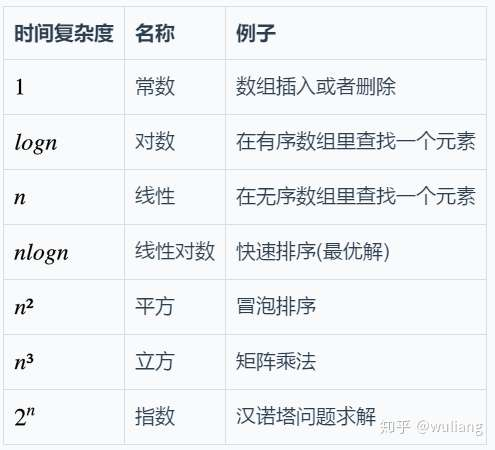# 数据结构和时间复杂度简单入门

## 数据类型

• 编程语言定义的数据类型(基本数据类型)
• 用户定义的数据类型

• boolean (布尔类型, 用于判断) (true false)
• byte (8位有符号整数)(最小值-128,最大值127)
• short (16位有符号整数)(最小值-32768, 最大值32767)
• int (32位有符号整数)(最小值-2147483648 (-2^31), 最大值2147483647 (2^31-1))
• long (64位有符号整数)(最小值-2^63 最大值2^63-1)
• float (32位浮点数)(1.4E-45~3.4028235E38)
• double (64位浮点数)(4.9E-324~1.7976931348623157E308)
• char (16位Unicode字符)

## 用户定义的数据类型

C/C++的结构体, Java的类

``````Java写法
public class Dog
{
byte age;
int birthday;
}
``````

## 时间复杂度## 例子

``````//循环执行n次
for(int i = 1; i<=n; i++)
{
int m = m+2; //时间常数c
}
``````

ex2

``````//循环了n次
for(int i = 1; i<=n; i++)
{
//循环了n次
for(int j = 1; j<=n; j++)
{
int m = m+2; //常数时间c
}
}
``````

if-then-else 条件语句: 最坏情况下的运行时间是: 条件判断时间+最大值(then运行时间或else运行时间)

ex1

``````int a = 10;
// 时间复杂度是O(n)
if(a == 10) //条件常数c
{
//循环了n次
for(int i = 0; i<a; i++)
{
System.out.println(i); //常数c
}
}
else 时间复杂度O(1)
{
System.out.println(a); //常数c
}
``````

##### 热门推荐
•浏览(1,004)
•浏览(597)
•浏览(492)
•浏览(482)
•浏览(390)
•浏览(389)
•浏览(377)
•浏览(367)
•浏览(363)
•浏览(359)